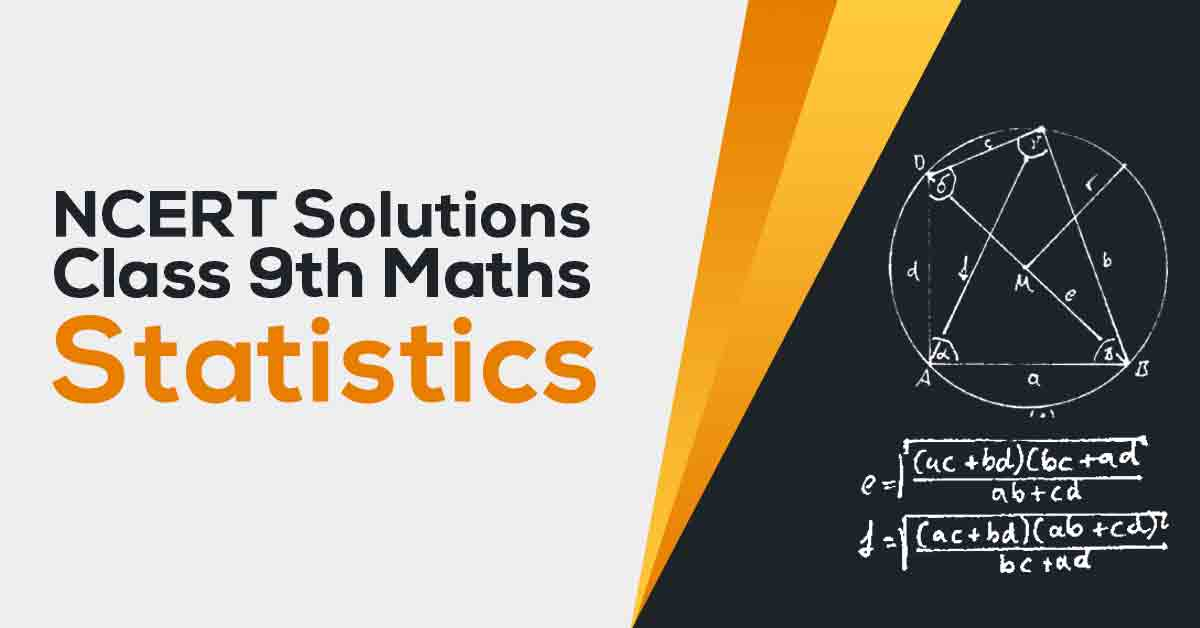NCERT Solutions for Class 9 Maths Chapter 14 | Vidyakul
×

# NCERT Solutions For Class 9 Maths Chapter 14

## NCERT Solutions For Class Maths Chapter 14 Statistics - PDF DownloadStudents find NCERT Solutions for Class 9 Maths Chapter 14 Statistics quite helpful for their Exam Preparation. In this chapter, you will learn Facts or figures, collected with a definite purpose, are called data. 2. Statistics is the area of study dealing with the presentation, analysis and interpretation of data. The three measures of central tendency for ungrouped data are: mean, median and mode.

These CBSE NCERT Solutions for Class 9 Maths Chapter 14 Statistics will help you to practice all the topics in the chapter with ease and score maximum marks in the examination.